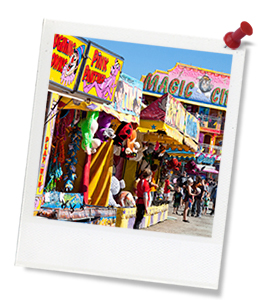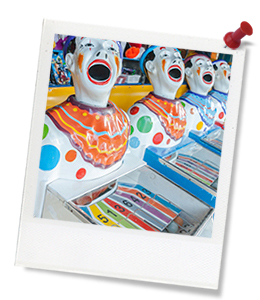## Activity IntroductionQuick summary: This lesson presents students with some explicit teaching around representing the probability of chance events from games (such as dice games) using fractions and decimals and placing the probability of given events on a probability number line. Following this, students conduct a simple chance experiment based on the outcome events of their ‘Sustainable STEM Sideshow Game’, collect event data and calculate the probability and event frequency of achieving a win in their Game.

This lesson sequence will require two standard classroom lessons of 45-50 minutes to complete.

Learning intentions:

• Students will describe events that are impossible and events that are certain
• Students will use the term ‘probability’ to describe the numerical value that represents the likelihood of an outcome of a chance experiments
• Students will use the term ‘frequency’ to describe the number of times a particular outcome occurs of a chance experiment
• Students will use the term ‘event’ to describe either one outcome or a collection of outcomes in the sample space of a chance experiment
• Students will represent and order probabilities of outcomes of chance experiments using fractions and decimals, e.g. ‘Equally likely’ would be placed at ½ or 0.5.

21st century skills:### Australian Curriculum Mapping

Content descriptions:

Year 5 to 8 Mathematics:

• Recognise that probabilities range from 0 to 1 (ACMSP117)
• Conduct chance experiments with both small and large numbers of trials using appropriate digital technologies (ACMSP145)
• Compare observed frequencies across experiments with expected frequencies (ACMSP146)
• Assign probabilities to the outcomes of events and determine probabilities for events (ACMSP168)

Syllabus outcomes: MA3-19SP

General capabilities: Numeracy, Creative and Critical Thinking

Relevant parts of Year 6 and 8 achievement standards:

By the end of Year 6, students compare observed and expected frequencies and they interpret and compare a variety of data displays including those displays for two categorical variables. Students add, subtract and multiply decimals and divide decimals and they describe probabilities using simple fractions, decimals and percentages.

By the end of Year 8, students explain issues related to the collection of data and they use efficient mental and written strategies to carry out the four operations with integers. They solve everyday problems involving percentages and they solve problems involving profit and loss.
Students choose appropriate language to describe events and experiments determine the probabilities of complementary events and calculate the sum of probabilities.

Topic: STEM, Mathematics, Sustainability

This lesson is part of the wider unit of work: Sustainable STEM Sideshow.

Time required: 90 mins (two 45-50 min lessons)

Level of teacher scaffolding: High – Teacher needs to explain various mathematical concepts around probability and assist students to apply the outcomes and event frequencies in their own Sideshow Game to the mathematical conceptual framework around probability (see below).

Resources required:

• Calculators – per student (for calculating decimal probability ratios)
• Dice (either digital and projected on a data projector or whiteboard, or large dice that the whole class can see)
• Interactive whiteboard or data projector
• Student Worksheet for Lesson 2: Probability (one per student)
• Students’ ‘Sustainable STEM Sideshow Game’ prototypes, from Lesson 1 of this Unit – set up ready to ‘test’ in a tabloid arrangement in the classroom or learning space
• Whiteboard (or electronic whiteboard) with pens.

Keywords: Chance, probability, likelihood, impossible, certain, even chance, event, outcome, frequency.

Cool Australia’s curriculum team continually reviews and refines our resources to be in line with changes to the Australian Curriculum.

##Teacher Preparation

Learning intentions: Students will...

• ... observe simple games and everyday events to describe them in terms of probability
• ... assign numerical values to events representing the likelihood of an outcome
• ... calculate a probability test for a game
• ... describe the number of times an outcome occurs in chance experiments using the term 'frequency'
• ... calculate the probability of events using fractions and decimals.

Success criteria: Students can…

• ... describe events that are impossible and events that are certain
• ... use the term 'probability' to describe the numerical value that represents the likelihood of an outcome of a chance experiment
• ... use the term 'frequency' to describe the number of times a particular outcome occurs in a chance experiment
• ... use the term 'event' to describe either one outcome or a collection of outcomes in the sample space of a chance experiment
• ... represent and order probabilities of outcomes of chance expe
...

## Thought Starter: What are the chances of that?

1. Following ‘The Chance Game’ that you play with your class at the start of this lesson, discuss these questions with your class and then note down the things that you SEE, THINK and WONDER about the probability of different events that you discussed in the game:

• In the Chance Game, what was the likelihood or chance that you’d be knocked out on any given turn? How do you know?
• If you chose an even number in a turn, what is the chance the die would roll an even number? How do you know?
• If you were allowed to, and you chose a ‘7’ as a number, what would be the chances of being knocked out in a round? Why?
• What were your chances of being the last person standing? How do you know?
• Can you think of any events in this game that could be described as ‘certain’?
.
 SEE THINK WONDER2. To work out the chance or probability of a player winning your game, we’ll need to:

1. Decide what a ‘win’ is – when do pl
...